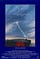# Outline:A movie called Short Circuit

A short-circuit evaluation of an expression is one in which the result is determined without evaluating all of the operands and/or operators.

• Example: (13 * a) * (b / 13–1)
• Explanation: the value of the arithmetic expression is independent of the value of (b / 13–1) if a is 0, because 0 * x = 0 for any x.
• Example: (a >= 0) && (b < 10)
• Explanation: The value of the Boolean expression, is independent of the second relational expression if a < 0, because the expression (FALSE && (b < 10)) is FALSE for all values of b
• Basically, short-circuit evaluation is dictates when the 1st part is 0(in 1st example) or false (in the 2nd example) evaluation of the rest is not necessary.
• Detection of shortcuts in arithmetic expressions is hard (1st example), however, it is easy in Boolean expressions (2nd one).

To illustrate a potential problem with non-short-circuit evaluation of Boolean expressions

• Example: suppose Java did not use short-circuit evaluation

index = 0;

while ((index < listlen) && (list[index] != key))

index = index + 1;

• Explanation: If evaluation is not short-circuit, both relational expressions in the Boolean expression of the while statement are evaluated, regardless of the value of the first. Thus, if key is not in list, the program will terminate with a subscript out-of-range exception. The same iteration that has index == listlen will reference list[listlen], which causes the indexing error because list is declared to have listlen-1 as an upper-bound subscript value.

A language that provides short-circuit evaluations of Boolean expressions and also has side effects in expressions allows subtle errors to occur.

• Example: (a > b) || ((b++) / 3)
• Explanation: In this expression, b is changed (in the second arithmetic expression) only when a <= b. If the programmer assumed b would be changed every time this expression is evaluated during execution (and the program’s correctness depends on it), the program will fail.

In the C-based languages, the usual AND and OR operators, && and ||, respectively, are short-circuit. However, these languages also have bitwise AND and OR operators, & and |, respectively, that can be used on Boolean-valued operands and are not short-circuit. Of course, the bitwise operators are only equivalent to the usual Boolean operators if all operands are restricted to being either 0 (for false) or 1 (for true).

# REFERENCES:

1. Sebesta, R. W. (n.d.). Expressions and Assignment Statements. In Concepts of Programming Languages (12th ed., pp. 319–323).